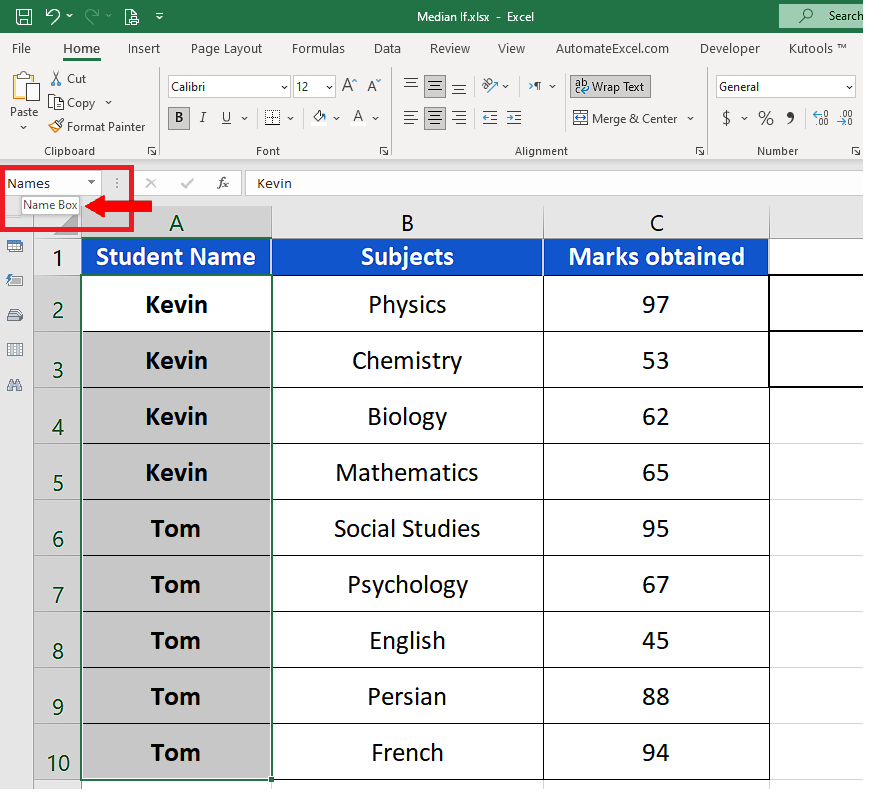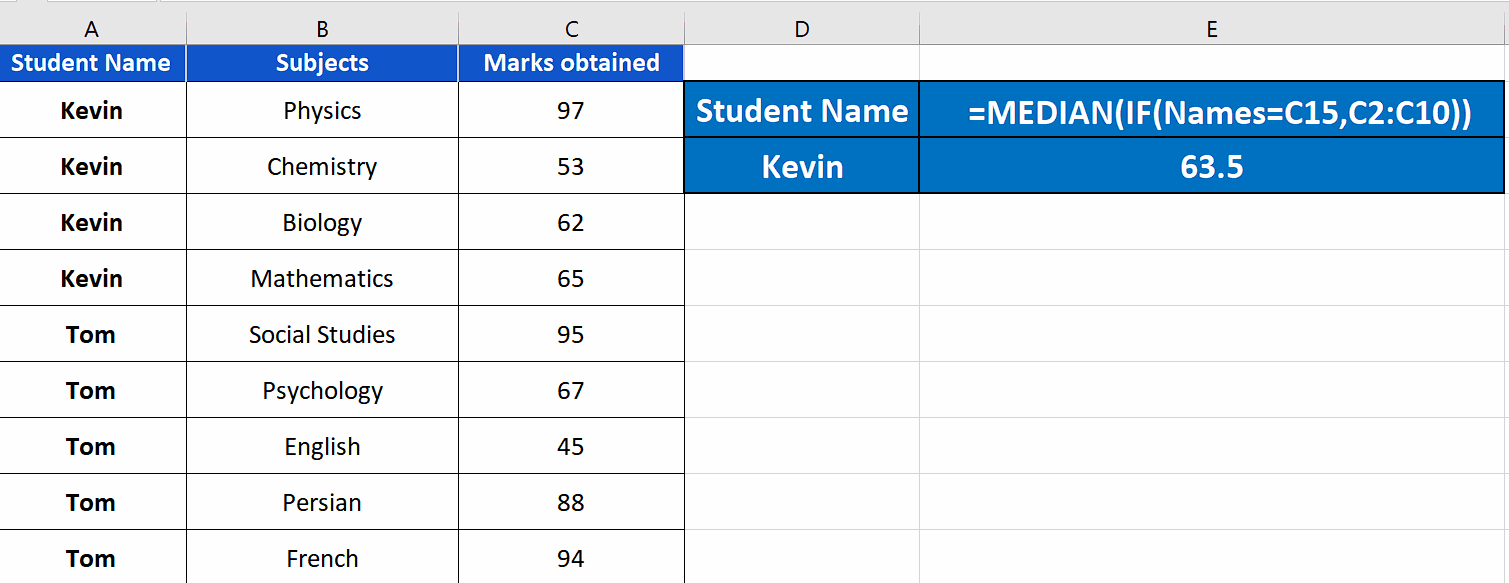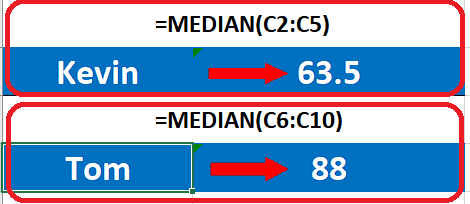# How to calculate Median based on a criteria (MEDIAN – IF)

When it comes to calculating the median of the data based on a criteria then Excel does not have the ability in the median function to achieve this goal. So to get it done, we need to use IF() along with MEDIAN(). In this tutorial, we’ll learn how to use the combination of these two functions to calculate the median of the data based on a special criteria.

Let’s see the data set for which we’ll calculate the median based on a criteria. Our data set consists of the details of the marks of two students and we wish to calculate the median of the marks obtained by a specific student whose name will be chosen in a specific cell.

So now we’ll calculate the median of the marks obtained by the student depending upon the name of the student.Excel provides us with a lot of functions and tools to analyse data sets. The nature of the data can vary and the type of analysis required on the data also varies with the nature of the data. Often we have to perform statistical analysis of the data and Excel has many standard functions for this purpose. One of the basic statistical functions is the MEDIAN(), which is used to calculate the median of the given data.

### Step 1 – Create a Named Range for the formula– We’ll create a named range consisting of the names of the students from the available data so that our formula can work properly.
– To create the named range, select all the names. Go to the Name Box and write Names. The text shown in the Name Box will be the name of the range of names from A2:A10.

### Step 2 – Create the appropriate formula using MEDIAN-IF– Now we can use the combination of both functions in the formula to get our desired results. The formula will be as follows;
=MEDIAN(IF(Names=D3,C2:C10))
– Our formula calculates the median of the scores of the particular student based on the name of the student present in the cell D3. We can see that the median of the score changes when the name of the student is changed.

### Step 3 – Manual Median– We can calculate the median of the same data manually to check whether our formula calculated the results properly or not. However, we’ll get the same answers.

## Breakdown of the formula:

We used the formula;

=MEDIAN(IF(Names=D3,C2:C10))

Let’s break down the formula from inside. The goal is achieved by using the IF function which checks for the value of cell D3 in the named range Names. When the value of cell D3 matches the name in the named range the corresponding data values are selected from the data range C2:C10. The output of the IF(Names=D3,C2:C10) as follow;

{97;53;62;65;FALSE;FALSE;FALSE;FALSE;FALSE}

When this array is passed on to the MEDIAN() function it calculates the median of the numeric values only and returns the correct value. Similarly in case of choosing Tom in D3, we’ll get the following array as the output of IF(Names=D3,C2:C10) and the MEDIAN() function will again calculate the median of the numeric values only.

{FALSE;FALSE;FALSE;FALSE;95;67;45;88;94}